## Introduction

The addition energy of an electron to a superconducting island, weakly coupled to the environment by the capacitance CΣ, is given by (see Methods):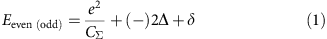where the first term is the Coulomb energy, the second term depends on the parity of electron occupation number as a consequence of the formation of a Cooper pair1,2 and the third term is the electronic level spacing in the island. This parity effect has been observed in large: 1 μm micro-fabricated Al islands, through direct measurement of the charge capacitance of the island2, through the even–odd modulation of the addition energy in single-electron transistors3,4,5,6 or the parity dependence of the Josephson current in Cooper pair transistors7,8,9.

Until now, the parity effect on the addition energy has never been observed in small nanocrystals (NCs) near the Anderson limit10, reached at a volume about VAnderson100 nm3, where the mean electronic level spacing <δ> equals the superconducting gap energy Δ.

In single-electron transistors fabricated with nanosized superconducting grains of aluminium11,12, the 2e modulation of the addition energy could not be observed directly. Also, because only a few devices could be fabricated, testing the Anderson criterion was not possible with this approach. Indirect indications for the disappearance of superconductivity in small superconducting grains came from magnetization measurements13,14; because these measurements were averaged over macroscopic quantities of NCs, the link to the Anderson limit remained ambiguous.

In this work, we present a new system that enables a study of single and isolated NCs across the Anderson limit, where the NCs can be reproducibly obtained in large quantities. The superconducting gap energy and the transition temperature are measured through a study of the superconducting parity effect in the addition energy of the NCs. This constitutes an alternative approach to conventional tunnelling measurement of the superconducting gap in the quasiparticle excitation spectrum, which cannot be accessed as a consequence of the Coulomb gap at zero bias.

## Results

### Sample preparation

The observation of the parity effect is challenging as it requires clean systems, free of impurity states responsible for the so-called quasiparticle poisoning9,15. Furthermore, scanning tunnelling spectroscopy of isolated NCs requires, in addition to the tip–NC tunnel barrier, a second tunnel barrier between the NC and the conducting substrate16,17, as sketched in Supplementary Fig. 1.

In this work, the Pb NCs are obtained by thermal evaporation of a nominal 0.3 monolayer of Pb on the (110) surface of InAs heated at T=150 °C. The (110) surface is obtained by cleaving an n-type InAs substrate in ultra-high vacuum at a base pressure P10−10 mbar. Two distinct samples (A and B) have been prepared with slightly different NC concentrations and sizes. The volume of the NCs ranges from 20 nm30.2 VAnderson to 800 nm38VAnderson while the height ranges from 1 unit cell (0.495 nm) to 5.2 nm, see Supplementary Fig. 2 and Supplementary Note 1 for details on NC volume determination. The scanning tunnelling microscopy (STM) topographic images (Fig. 1a–c; Supplementary Fig. 3) for sample A and sample B, respectively, show that Pb grows in the Volmer–Weber, that is, Island mode18. The three dimensional Laplacian image Δxyz(x,y) (Fig. 1c) shows that the NCs are well crystallized and expose mostly the (111) planes of the cubic face-centred Pb structure, as indicated by the observation of the characteristic hexagonal shape of the (111) facets. Surrounding these NCs, the surface remains free from adsorbate, as atomic resolution images of the (110) InAs surface prove (Fig. 1d).

### Tip-induced QDot on the InAs surface

Figure 1e shows the differential conductance (DC) dI/dV measured on the InAs surface at several distances, from 0 to 10 nm, of a Pb NC. The data are measured at T=1.3 K, unless indicated otherwise, using a standard lock-in procedure (see Methods). The data indicate that the Fermi level is in the conduction band of InAs as expected for this n-doped sample. With a sulphur dopant concentration, ND6 × 1016 cm−3, the Fermi level is 21 meV above the conduction band minimum. A zoom on these spectra (Fig. 1f) shows multiple peaks that result from the discrete levels of the tip-induced quantum dot (QDot), a phenomena that has also been observed in previous works19. This demonstrates that Pb deposition on InAs do not produce any significant defects and doping. Indeed, in the presence of defects or adsorbate, the surface of III–V semiconductors present interface states that pin the Fermi level at the charge neutrality level20,21 (Fig. 1g). For InAs, this level is located 150 meV above its conduction band minimum, which leads to the formation of an electron accumulation layer as shown by numerous photoemission experiments20,22. In contrast, perfectly clean (110) surfaces do not present any interface states and consequently the Fermi level is not pinned. Thus, the electric field from the STM tip can easily shift the conduction band and generates the so-called tip-induced QDot19, as sketched in Fig. 1h. While the energy of the QDot levels can shift on long distances, see Supplementary Fig. 4, as a consequence of variations in the electrostatic environment due to the random distribution of Pb NCs and sulphur dopants, we see (Fig. 1f) that the QDdot levels are not altered on short distances (<10 nm) near the NCs. Only a weak broadening of the QDot levels is observed, likely a consequence of their weak tunnel coupling with the Pb NCs.

### Coulomb blockade and nature of the tunnel barrier

On NCs of three distinct sizes shown in Fig. 2a–c, representative DC spectra are shown in Fig. 2d,e. They display a Coulomb gap at zero bias of width δVsub=e/(Csub+Ctip), where Csub (Ctip) is the capacitance between the NC and the substrate (tip). The data also display sharp Coulomb peaks where the voltage interval between the peaks provides the addition voltage δVadd for an electron, which is related to the addition energy by: δVadd=Eadd/, whereis the arm lever; see the Methods section for a derivation of these relations. Furthermore, the DCs may also display broad additional peaks, of weak amplitude in large NCs, V/VAnderson>1, as indicated by arrows in Fig. 2d, but of large amplitude in small NCs, V/VAnderson<<1, as indicated by arrows in Fig. 3. These broad peaks are the signature of quantum well states in the Pb NCs due to strong confinement in the <111> direction as observed in scanning tunnelling studies of thin layers of Pb23.

The colour map in Fig. 2e shows that δVadd changes slightly with the tip position above the NC, as consequence of the variation in the tip–NC capacitance Ctip. Figure 3 shows the DCs for 13 additional NCs, from which the capacitance Csub is extracted and shown as coloured symbols in Fig. 2f and Supplementary Fig. 5. On these last plots, data points shown as black circles of 24 other NCs are also included, for which the DCs are not shown. Figure 2f shows that Csub increases linearly with the area A as Csub=/d, using ɛ=12.3, the dielectric constant of InAs and d=4 nm for the effective tunnel barrier thickness.

As no dielectric insulator has been deposited on the surface and no Schottky barrier exists at metal–InAs interfaces21,22, the origin of the tunnel barrier and the meaning of the thickness d appear clearly only after one realizes that the Fermi wavelength of the two-dimensional (2D) gas in InAs is larger than the lateral size of the NCs. At the interface between the Pb NC and InAs, the Fermi energy in InAs is at the charge neutrality level, EF=150 meV (refs 21, 22), which gives for the Fermi wavelength λF=20 nm. As known from numerous works with quantum point-contacts formed in 2D electron gas24,25, the transmission coefficient T decreases for constrictions smaller than the Fermi wavelength. Because a NC covers only a fraction of the area, its transmission coefficient with the 2D gas is significantly smaller than one, which explains the observation of the Coulomb blockade. For a small NC, the weak coupling model26 can be used to describe the data, as shown in Fig. 2g. This model shows that the contact impedance is of the order of Rcontact10 MΩ, implying that the transmission coefficient T=Rcontacte2/h=0.0025 is weak as anticipated. In this model, the magnitude of the Coulomb peaks increases with the ratio Rtunnel/Rcontact, as observed on the DC curves measured as function of tip height (Supplementary Fig. 6). Figure 2h shows the amplitude of the Coulomb peak, normalized to its base value, as function of NC area. The amplitude is constant for small area (<100 nm2) but decreases quickly for area approaching π/4300 nm2. This behaviour cannot be described by the weak coupling model just discussed; however, it can be understood by considering models of Coulomb blockade in the strong coupling regime27,28. These models show that the Coulomb oscillations disappear when T approaches unity, when charge fluctuations between the NC and the substrate become significant. Figure 3 shows that the Coulomb peaks of the largest NCs have almost completely disappeared. The fact that the amplitude of the Coulomb peaks decreases for NCs area approaching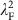confirms our interpretation that the tunnel barrier is due to a quantum constriction of the electronic wave function at the interface between the NC and the 2D gas. Thus, the dielectric thickness d=4 nm extracted from Csub above is actually set by the Debye length of the 2D gas and Csub actually corresponds to the quantum capacitance of InAs.

### Superconducting parity effect

For NCs smaller than the Anderson volume (Fig. 4d,g,j), we observe that δEHT is non-zero, which indicates that the electronic level spacing δ has now a significant contribution to the addition energy, following equation (1). The values of δEHT are distinct between successive charge configurations. Indeed, in metallic systems, the electronic levels are randomly distributed as described by random matrix theory (RMT)29. Collecting the values δEHT for all NCs, Fig. 5a shows that, in average, the evolution of δEHT with NC volume can be properly described by the relation: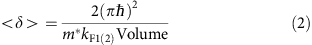using m*=1.2 me for the effective mass, where kF1=7.01 nm−1 and kF2=11.21 nm−1 are characteristic wavevectors of the two Fermi surfaces FS1 and FS2 of Pb.

For the NC of volume V/VAnderson=0.89 (Fig. 4d), while the level spacing δEHT is large, the shift of the Coulomb peaks due to the parity effect is still dominating the temperature dependence and can be observed directly on the raw data and the addition energy difference δE plotted as the function of temperature in Fig. 4e. A line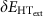is extrapolated from high temperature and the difference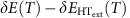gives the temperature dependence of the superconducting gap (Fig. 4f), which shows that the critical temperature Tc6 K is smaller than the bulk value. The amplitude of the superconducting gap is obtained from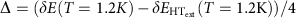. For this NC, the superconducting energy gap is about two times smaller than the bulk value, Δ=Δbulk/2.

For the smaller NC of volume V/VAnderson=0.55 (Fig. 4g), the level spacing δEHT is larger and has a temperature dependence that dominates the shift of the Coulomb peaks with temperature. This shift could be the consequence of thermally induced electrochemical shifts or temperature-dependent strain or electric field effects. While the parity effect is barely visible on the raw data, using the procedure employed for the previous NC, the temperature Tc5 K value and the energy gap ΔΔbulk/4 can be extracted (Fig. 4i).

Finally, for the smallest NCs V/VAnderson=0.43 (0.34), shown, respectively, in Fig. 4j and Supplementary Fig. 8h, they have the largest level spacing δEHT and, even though the addition energies are measured with much higher resolution than the superconducting gap energy, no parity effect can be observed in Fig. 4l and Supplementary Fig. 8j, respectively.

For 13 NCs where the DCs have been acquired as the function of temperature, some of which are shown in Fig. 4 and Supplementary Fig. 8, the level spacing, the superconducting gap energy and the transition temperature are extracted and plotted (Fig. 5a–c), respectively. Upon reducing the NC volume, both quantities display a sharp decrease to zero when the level spacing becomes of the order of the superconducting gap energy, 1 meV. See Supplementary Note 2 for a comparison with the results of Bose et al.17 on a system where the superconducting nanoparticles are strongly coupled to the normal substrate.

## Discussion

Figure 5 suggests that superconductivity disappears when the mean level spacing at the Fermi surface of the electron-type band (Fig. 5e), increases up to the superconducting gap energy. This is consistent with recent theoretical calculations30 and STM measurements31, which have shown that electron–phonon coupling is stronger for this electron-type band owing to its p–d character. Regarding the Bardeen-Cooper-Schrieffer ratio kBTc/Δ, within the experimental resolution, no significant deviation from the bulk value has been observed.

To summarize, we have found that a 2D electron gas of large Fermi wavelength constitutes an ideal substrate for studying Coulomb blockade in nanosized NCs evaporated in an ultra-high vacuum environment. This discovery leads us to observe, for the first time by STM, the parity effect and quantum confinement in isolated superconducting NCs and enabled the first demonstration of the Anderson criterion for the existence of superconductivity at single NC level. Furthermore, this new insight on the superconductor–InAs interface is of interest for topological superconductivity where Majorana islands are generated by depositing a superconductor on InAs nanowires32,33.

## Methods

### Relation between sample bias and energies

The Coulomb gap at zero bias results from Coulomb blockade that prevent charge fluctuations in the NC. As sketched in Supplementary Fig. 1, Coulomb blockade is lifted when the Fermi level of either one of the electrodes is aligned with one of the excited levels of the NC. Thus the amplitude of the Coulomb gap observed in the DC is given by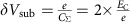, with.

The Coulomb peaks observed at higher voltages result from the shift of the electrochemical potential of the NC upon increasing the voltage bias across the double junction. This shift is given by:with: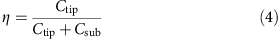Charge states with increased number of electrons become accessible when the electrochemical potential changes by 2 × EC. Thus, the voltage difference between two charge states is given by: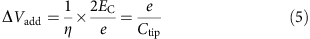This formula shows that the addition voltage depends only on the capacitance Ctip and not on the capacitance Csub, as shown in Supplementary Fig. 1b, where a simulation of the conduction spectrum, using the Hanna and Tinkham model26 for two distinct values of the capacitance Csub.

Following refs 1, 34, the total energy of a NC with N electrons is given by: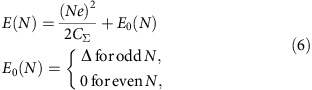The electrochemical potential of a NC with an even (odd) N (N+1) number of electrons is given by: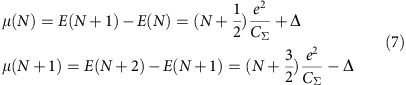From these last equations, one obtains the addition energies for a NC with an even (odd) N (N+1) number of electrons: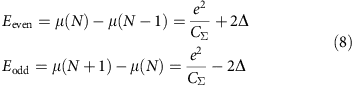Thus, the difference of addition energies between two successive charge states is given by: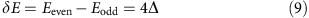When the electronic spectrum of the NC is discrete, the level spacing δ should be included in the addition energy.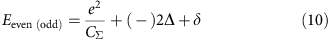### Random level distribution

In metallic NCs, the electronic level distribution is described by RMT29,35. In a NC with strong spin–orbit coupling, RMT predicts that the level spacing should be described by a Gaussian symplectic ensemble. For this level distribution, shown in Supplementary Fig. 9, the width of the distribution, that is, the s.d., is equal to σ<δ> (refs 29, 36). Between two successive charge states, the addition energy can fluctuate by an amount of the order of σ, consequently, in average, the difference in addition energies between two successive charge states is given by:At temperatures above the superconducting transition temperature: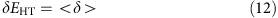Thus, an estimation of the level spacing can be obtained by a measure of the difference in the addition energies above TC.

Furthermore, the gap amplitude can be obtained from: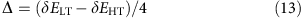### Measurements details

The microscope used is a low temperature, Tbase=1.3 K, Joule–Thomson STM from SPECS accommodated with a preparation chamber operating in ultra-high vacuum at a base pressure P10−10 mbar. The DC curves dI/dV are measured with a standard lock-in procedure. An a.c. signal of amplitude 1 meV and frequency 777 Hz is employed.

### Data avaibility

The data that support the main findings of this study are available from the corresponding author upon request.